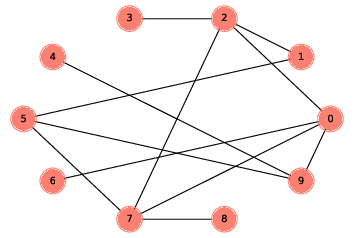# 4.7. Generating ER Graphs¶Figure 4.4: An ER graph with n=10 and p=0.3.

The ER graph $$G(n, p)$$ contains $$n$$ nodes, and each pair of nodes is connected by an edge with probability $$p$$. Generating an ER graph is similar to generating a complete graph.

The following generator function enumerates all possible edges and chooses which ones should be added to the graph:

def random_pairs(nodes, p):
for edge in all_pairs(nodes):
if flip(p):
yield edge


random_pairs uses flip:

def flip(p):
return np.random.random() < p


This is the first example we’ve seen that uses NumPy. Following convention, we will import numpy as np. NumPy provides a module named random, which provides a method named random, which returns a number between 0 and 1, uniformly distributed.

So flip returns True with the given probability, p, and False with the complementary probability, 1-p.

Finally, make_random_graph generates and returns the ER graph $$G(n, p)$$:

def make_random_graph(n, p):
G = nx.Graph()
nodes = range(n)

make_random_graph is almost identical to make_complete_graph; the only difference is that it uses random_pairs instead of all_pairs.
Here’s an example with $$p=0.3$$:
random_graph = make_random_graph(10, 0.3)

Figure 4.4 shows the result. This graph turns out to be connected; in fact, most ER graphs with $$n=10$$ and $$p=0.3$$ are connected. In the next section, we’ll see how many.